# 双指针技巧

## 相关程序练习

### 1. Reverse String(反转字符串）

• 编写一个函数，其作用是将输入的字符串反转过来。输入字符串以字符数组 char[] 的形式给出。
• 不要给另外的数组分配额外的空间，你必须原地修改输入数组、使用 O(1) 的额外空间解决这一问题。
• 你可以假设数组中的所有字符都是 ASCII 码表中的可打印字符。

Input: [“h”,“e”,“l”,“l”,“o”]
Output: [“o”,“l”,“l”,“e”,“h”]

#### 实现代码

``````void reverseString(char* s, int sSize){
int front=0, back=sSize-1;
while(front<back){
char temp;
temp = s[front];
s[front] = s[back];
s[back] = temp;
front++;back--;
}
return s;
}``````

#### 执行结果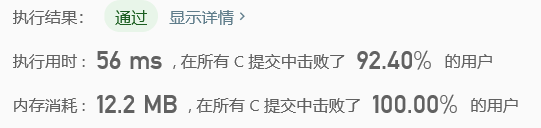### 2. 数组拆分 Array Partition I

#### 算法：鸡尾酒算法plus

``````int arrayPairSum(int* nums, int numsSize){
bool isSorted = true;
int lastLeftIndex=0, lastRightIndex=0;
int leftBorder = 0, rightBorder = numsSize - 1;
for(int i=0; i<numsSize/2; i++){
for (int j = leftBorder; j < rightBorder; j++) {
if (nums[j] > nums[j + 1]) {
int temp = nums[j];
nums[j] = nums[j + 1];
nums[j + 1] = temp;
lastRightIndex = j;
isSorted = false;
}
}
rightBorder = lastRightIndex;
if (isSorted) break;

for (int j = rightBorder; j > leftBorder; j--) {
if (nums[j] < nums[j - 1]) {
int temp = nums[j];
nums[j] = nums[j - 1];
nums[j - 1] = temp;
lastLeftIndex = j;
isSorted = false;
}
}
leftBorder = lastLeftIndex;
if (isSorted)  break;
}
for(int i=0; i<numsSize; i++) printf("%d  ",nums[i]); printf("\n");
int sum=0;
for(int index=0; index<numsSize; index++){
if(!(index%2)) sum += nums[index];
}
return sum;
}    ``````

#### 算法：使用qsort函数

``````int comp(const void* a, const void* b){
return (*(int*)a > *(int*)b);
}
int arrayPairSum(int* nums, int numsSize){
qsort(nums, numsSize, sizeof(int), comp);
int sum = 0;
for(int i=0;i<numsSize;i+=2){
sum += nums[i];
}
return sum;
}``````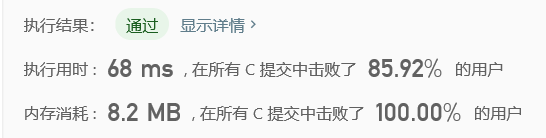### 3. 两数之和II-输入有序数组

#### 法一：暴力解决

``````int* twoSum(int* numbers, int numbersSize, int target, int* returnSize){
if(numbers[i] + numbers[j] == target){
* returnSize = 2;
* int* two = (int *)malloc(sizeof(int) * 2);
two = i+1; two = j+1;
return two;
}
}
}
*returnSize = 0;
return NULL;
}``````

#### 法二：双指针

``````int* twoSum(int* numbers, int numbersSize, int target, int* returnSize){
int* two = (int *)malloc(sizeof(int) * 2);
while(low<high){
int sum = numbers[low] + numbers[high];
if(sum==target){
two = low + 1;
two = high + 1;
* returnSize = 2;
return two;
}
else if(sum<target) low++;
else high--;
}
*returnSize = 0;
return NULL;
}``````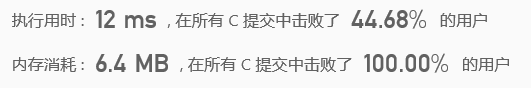## 相关程序练习

### 1. 移除元素

#### 法一：交换

``````int removeElement(int* nums, int numsSize, int val){
int low=0, high=numsSize-1, size=numsSize;
while(low<high){
if(nums[low]!=val) low++;
else if(nums[low]==val){
while(nums[high]==val){
high--;
size--;
if(high==low) return low;
}
int temp = nums[low];
nums[low] = nums[high];
nums[high] = temp;
size--;
low++;
high--;
}
}
if(high==low && nums[low]==val) size--;
return size;
}``````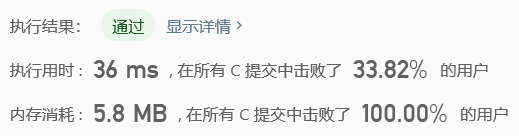#### 法二：就题论题，因题制宜

``````int removeElement(int* nums, int numsSize, int val){
int k=0;
for (int i=0;i<numsSize;i++){
if (nums[i]!=val){
nums[k] = nums[i];
k++;
}
}
return k;
}``````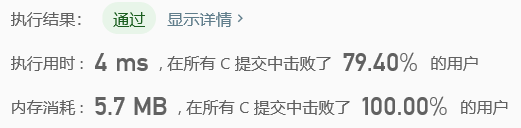### 2. 最大连续1的个数

``````int findMaxConsecutiveOnes(int* nums, int numsSize){
int max=0;
for(int i=0; i<numsSize; i++){
int n=0;
while(i+n<numsSize && nums[i+n]) n++;
i += n;
if(n) max = max>n?max:n;
}
return max;
}``````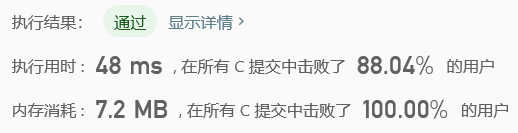### 3. 长度最小的子数组

#### 法一：暴力解决(也不是很暴力那种)

``````int minSubArrayLen(int s, int* nums, int numsSize){
if(numsSize < 1) return 0;
int min = numsSize;
bool flag=false;
for(int i=0; i<numsSize; i++){
int sum=0, n=0;
while(i+n<numsSize && sum<s) sum+=nums[i+(n++)];
if(sum>s-1){
flag=true;
min = min<n?min:n;
}
}
if(flag) return min;
else return 0;
}``````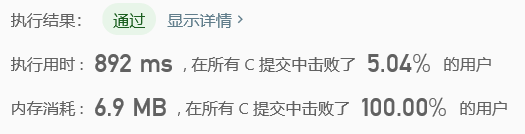#### 法二：双指针(窗口滑动)

``````# define MIN(a,b) a<b?a:b

int minSubArrayLen(int s, int* nums, int numsSize){
if(numsSize < 1) return 0;
int min = INT_MAX , left = 0 , sum = 0;
for(int i = 0 ; i < numsSize ; i++){
sum += nums[i];
while(s <= sum){
min = MIN(min , (i  - left + 1));
sum -= nums[left++];
}
}
return min == INT_MAX ? 0 : min;
}``````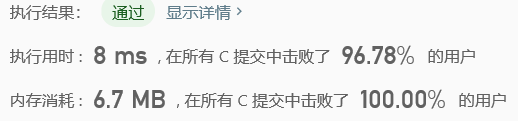#### 小技巧

``# define MIN(a,b) a<b?a:b``

``````int min(int a, int b){
return a<b?a:b;
}``````